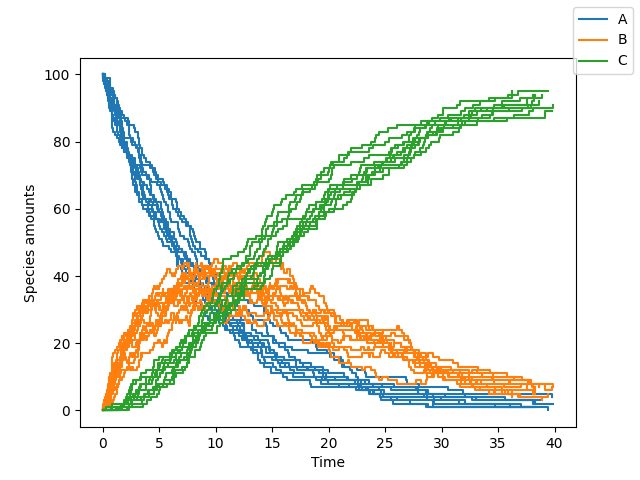Logo for cayenne

# cayenne : Python package for stochastic simulations¶

## Introduction¶

cayenne is a Python package for stochastic simulations. It offers a simple API to define models, perform stochastic simulations with them and visualize the results in a convenient manner.

Currently under active development in the develop branch.

## Install¶

Install with pip:

\$ pip install cayenne


## Usage¶

A short summary follows, but a more detailed tutorial can be found here. You can define a model as a Python string (or a text file, see docs). The format of this string is loosely based on the excellent antimony library, which is used behind the scenes by cayenne.

from cayenne.simulation import Simulation
model_str = """
const compartment comp1;
comp1 = 1.0; # volume of compartment

r1: A => B; k1;
r2: B => C; k2;

k1 = 0.11;
k2 = 0.1;
chem_flag = false;

A = 100;
B = 0;
C = 0;
"""
# Run the simulation
sim.simulate(max_t=40, max_iter=1000, n_rep=10)
sim.plot()Plot of species A, B and C

### Change simulation algorithm¶

You can change the algorithm used to perform the simulation by changing the algorithm parameter (one of "direct", "tau_leaping" or "tau_adaptive")

sim.simulate(max_t=150, max_iter=1000, n_rep=10, algorithm="tau_leaping")


Our benchmarks are summarized below, and show direct to be a good starting point. tau_leaping offers greater speed but needs specification and tuning of the tau hyperparameter. The tau_adaptive is less accurate and a work in progress.

### Run simulations in parallel¶

You can run the simulations on multiple cores by specifying the n_procs parameter

sim.simulate(max_t=150, max_iter=1000, n_rep=10, n_procs=4)


### Accessing simulation results¶

You can access all the results or the results for a specific list of species

# Get all the results
results = sim.results
# Get results only for one or more species
results.get_species(["A", "C"])


You can also access the final states of all the simulation runs by

# Get results at the simulation endpoints
final_times, final_states = results.final


Additionally, you can access the state a particular time point of interest $$t$$. cayenne will interpolate the value from nearby time points to give an accurate estimate.

# Get results at timepoint "t"
t = 10.0
states = results.get_state(t) # returns a list of numpy arrays


## Benchmarks

cayenne :he avy_check_mark: Most accurate yet :he avy_check_mark: Very fast but may need manual tuning Less accurate than GillespieSSA’s version
Tellurium

Inaccurate for 2nd order

N/A N/A
GillespieSSA Very slow

Inaccurate for initial zero counts

Inaccurate for initial zero counts

BioSimulator.jl

Inaccurate interpolation

Inaccurate for initial zero counts

Inaccurate for initial zero counts